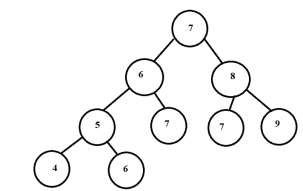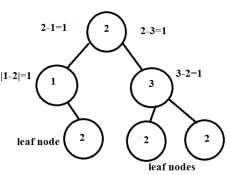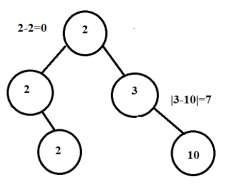# Continuous Tree in C++

A Continuous Tree is defined as a tree with any path from root node to leaf node has value or weight of nodes such that the absolute difference between the parent node and all of its direct children nodes is always 1.

If we pick any node on the path from root to leaf, then

|weight of node-weight of left child node|=|weight of left child node-weight of node| = 1, this holds true for right child as well

|weight of node-weight of right child node|=|weight lof right child node-weight of node| = 1

DiagramLet us understand with examples.

The tree below is continuous as absolute difference between parent nodes and their child is always 1.The tree below is not qualified for being a Continuous Tree.## Algorithm to Find if tree is Continous

• If root is NULL, return 1

• If it is a leaf node, return 1 as the tree has been Continuous that's why the leaf node reached.

• If the left subtree is empty check continuity of the current node with the right child ( calculate absolute difference of weights) and continue for the right subtree recursively.

• If the right subtree is empty check continuity of the current node with the left child ( calculate absolute difference of weights) and continue for the left subtree recursively.

• Else calculate absolute difference with weights of left and right child and continue for left and right subtrees, recursively.

## Pseudocode

// Function to check tree is continuous or not
struct btreeNode{
int data;
btreeNode* left, * right;
};
int isContinuous(btreeNode *root){
// if node is NULL return 1, exit condition
if (root == NULL)
return 1;
//if leaf node is reached then tree must be continous during this path
if (root->left == NULL && root->right == NULL)
return 1;
// if no left child
if (root->left == NULL)
return (abs(root->data - root->right->data) == 1) && isContinuous(root->right);
// if no right child
if (root->right == NULL)
return (abs(root->data - root->left->data) == 1) && isContinuous(root->left);
// calculate absoute difference
return abs(root->data - root->left->data)==1 && abs(root->data - root->right->data)==1 &&
isContinuous(root->left) && isContinuous(root->right);
}